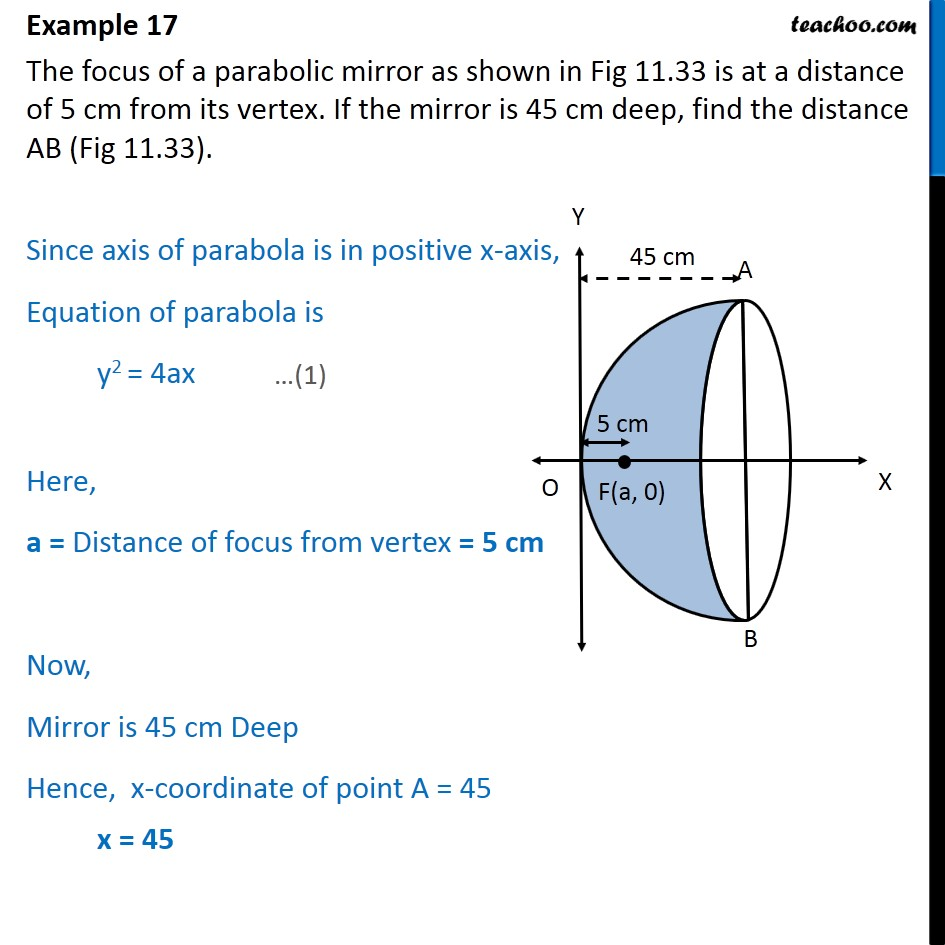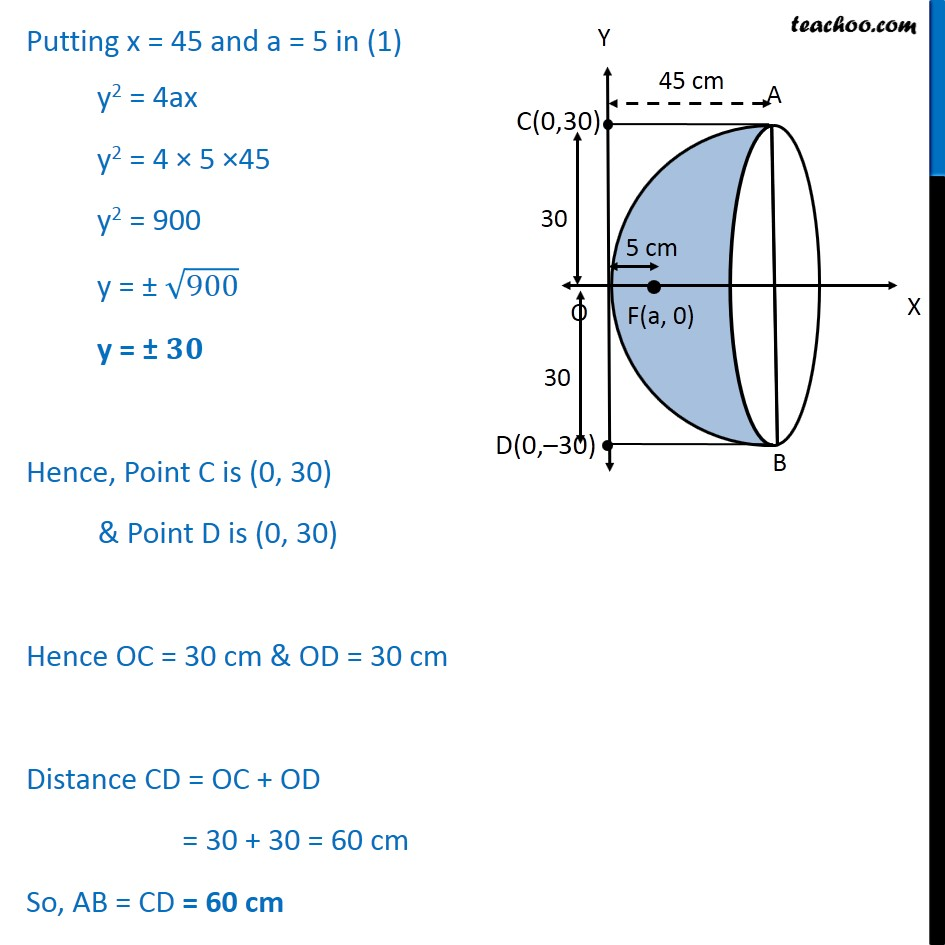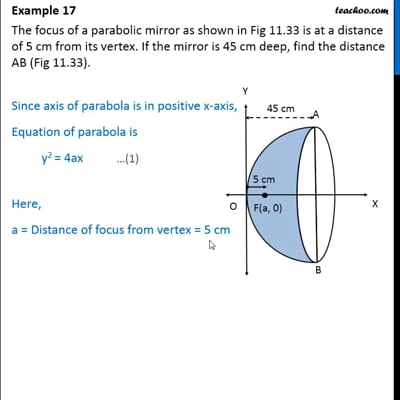Parabola - Arch/mirror problem

Chapter 11 Class 11 Conic Sections
Concept wiseThis video is only available for Teachoo black users

Introducing your new favourite teacher - Teachoo Black, at only ₹83 per month

### Transcript

Example 17 The focus of a parabolic mirror as shown in Fig 11.33 is at a distance of 5 cm from its vertex. If the mirror is 45 cm deep, find the distance AB (Fig 11.33). Since axis of parabola is in positive x-axis, Equation of parabola is y2 = 4ax Here, a = Distance of focus from vertex = 5 cm Now, Mirror is 45 cm Deep Hence, x-coordinate of point A = 45 x = 45 Putting x = 45 and a = 5 in (1) y2 = 4ax y2 = 4 × 5 ×45 y2 = 900 y = ± ﷐﷮900﷯ y = ± 𝟑𝟎 Hence, Point C is (0, 30) & Point D is (0, 30) Hence OC = 30 cm & OD = 30 cm Distance CD = OC + OD = 30 + 30 = 60 cm So, AB = CD = 60 cm